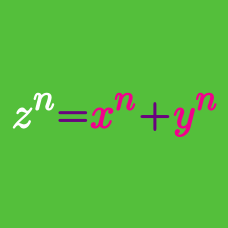Number Theory

# General Diophantine Equations: Level 4 Challenges

Note that $7!=10 \times9 \times8\times7.$

What is the largest possible value of $n$ for which $n!$ can be represented as a product of $n-3$ consecutive integers?

How many ordered pairs of positive integers $(A,B)$, each of which are between 1 and 100 inclusive, are there such that

$A^B = B^A ?$

I choose two different integers, both greater than 1, whose sum is less than 100. I whisper this sum to Sam, and whisper their product to Paula. They then have the following conversation:

Paula: I don’t know the numbers.

Sam: I knew you didn’t. I don’t either.

Paula: Ah, now I know them.

Sam: Now I know them too.

What is the LARGER number that I chose?

Credit for this problem goes to Hans Freudenthal in 1969 -- but do not Google it yet or you will have the solution spoiled!Positive integers are written on all faces of a cube, one on each. At each corner of the cube, the product of the numbers on the faces that meet at the corner is written. The sum of the numbers written at all the corners is $2004$. If $T$ denotes the sum of the numbers on all faces, find the sum of all possible values of $T$.

$\left( 1+\dfrac1a\right)\left( 1+\dfrac1b\right)\left( 1+\dfrac1c\right)\left( 1+\dfrac1d\right)\left( 1+\dfrac1e\right) = 14.4$

Find the number of 5-tuples of positive integers $(a, b, c, d, e)$ that satisfy the equation above.

×

Problem Loading...

Note Loading...

Set Loading...